IEX Resin
Posted in

# Ion Exchange Reactions

This article shows the general concept of ion exchange equilibrium, selectivity coefficients, and typical reactions of the different types of ion exchange resins.

### Equilibrium

An ion exchange resin in the ionic form A is in contact with a solution containing an ion B, an equilibrium reaction is observed. Here an example of cation exchange:

(1)

R-A+ + B+ <—–> R-B+ + A+

Ions A will migrate into the solution and be replaced by ions B from the solution until equilibrium is reached in the ion exchange process. The mass action law describes this equilibrium as follows:

(2)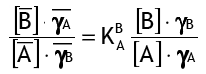where [..] represents concentrations and activity coefficients, and the bar represents the resin phase. For the ion exchange reaction, K is the selectivity coefficient of B+ over A+:

(3)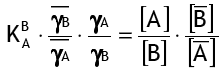To begin with, the activity coefficients are assumed to be constant, so the simplified selectivity coefficient is:

(4)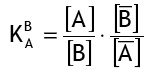Equation (1) becomes: for the most recent reaction between a strongly acidic resin in the H+ form and sodium ions (Na+) in water.

(5)

R-H+ + Na+ <—–> R-Na+ + H+

And the corresponding selectivity coefficient is

(6)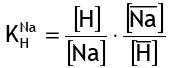For a standard, gel type sulphonic strongly acidic exchange resin, the coefficient K(Na/H) is around 1.7 (see the table of selectivity coefficients). This means that if a resin with a known Na and H ion composition is immersed in pure water, sodium ions will migrate into the water until the sodium concentration reaches the equilibrium determined by equation (6).

For divalent ions, e.g. (softening reaction):

(7)

2 R-Na+ + Ca++ <—–> R2-Ca++ + 2 Na+

The selectivity coefficient is:

(8)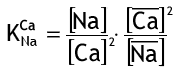Regeneration

Regeneration is the process of reversing the equilibrium and increasing the concentration of the displaced ion in the equilibrium reaction. For example, on the right side of the reaction, a softener is regenerated by using a high concentration of [Na+] ions (7). As a result, the reaction is shifted to the left.

Column operation

In normal ion exchange practice, the resin is not left in equilibrium with the solution. Instead, the solution is withdrawn at the column’s outlet, shifting the equilibrium to the right indefinitely. Examine equation (1) once more:

(1)

R-A+ + B+ <—–> R-B+ + A+

The treated solution, which is enriched in ions A+ and depleted in ions B+, is carried away and replaced by untreated solution, which has a low concentration of ions A+ but a high concentration of [B+]. Because the equilibrium is shifted to the right, the resin can remove more B+ ions from the solution.

Let us now look at the specific behaviour of common ion exchange resins.

### Strongly acidic resins

When the resin is in the hydrogen form H+ (R-SO3-H+, abbreviated R-H), it can remove all cations from solution:

(9)

R-H + Na+Cl <—–> R-Na + H+Cl

Second example with calcium bicarbonate:

(10)

2 R-H + Ca++(HCO3)2 <—–> R2-Ca + 2 H2CO3

Because the bicarbonate anion is alkaline, the reaction is a neutralisation. It is irreversible because a SAC resin cannot be regenerated with carbonic acid.

Reactions (9) and (10) are common as a first demineralisation step. See decationisation.

SAC resins remove divalent cations from water when the resin is initially in the sodium form Na+, but not other monovalent cations, because the selectivity difference with K+ or NH4+, for example, is too small.

(11)

2 R-Na + Ca++(HCO3)2 <—–> R2-Ca + 2 Na+HCO3

This softening reaction is reversible, but because of the risk of calcium carbonate precipitation, you would not regenerate with sodium bicarbonate. Sodium chloride is used instead.

### Weakly acidic resins

Because they are only weakly ionised in regenerated (H+) form, they react differently. There is no reaction with neutral salts:

(12)

R-COOH + Na+Cl <—–> nothing

Here’s an explanation: assuming the reaction occurs, it will produce HCl as a byproduct. However, because the resin is a weaker acid than the hydrochloric acid product, it would immediately revert to its acidic state.

The weak acid resin must be dissociated before it can react. As a result, the H+ ion in the acid must be removed by an alkali. For example, the following is an immediate and irreversible reaction:

(13)

R-COOH + Na+OH <—– R-COONa+ + H+OH

This is a neutralisation reaction in which the product is water (hence the irreversibility).

In the following reaction:

(14)

2 R-COOH + Ca++(HCO3)2 —–> (R-COO)2Ca++ + 2 [H+HCO3]

The bicarbonate ion “rips off” the H from the carboxylic group, causing it to be ionised. In water treatment, weak acid cation resins are used to remove bicarbonate hardness. This is the process of de-alkalisation. See the resin structure page for the complex formed. The reaction occurs with sodium bicarbonate instead of calcium or magnesium bicarbonate, but the WAC resin has much less affinity for monovalent ions, so the operating capacity is low:

(15)

R-COOH + Na+HCO3 —–> R-COONa+ + H+HCO3

Once converted to the Na+ form, divalent ions can be exchanged even in the absence of alkaline anions:

(16)

2 R-COONa+ + Ca+Cl2 —–> (R-COO)2Ca++ + 2 Na+Cl

Because of the high selectivity of these resins for hardness ions, softening with a WAC resin is very efficient. Because the residual hardness in the treated water is very low, the reaction (16) process is used to soften water in the presence of a high sodium concentration background. In practice, before being used for softening, the WAC resin is converted to the Na+ form with soda ash (Na2CO3) or caustic soda (NaOH).

Conclusions: When used normally (H-cycle, regeneration with HCl), the WAC resin removes only hardness and only when alkalinity is present; when used softening (Na-cycle), the WAC resin must first be converted to the Na form with an alkali.

### Strongly basic resins

When the resin is initially in the hydroxide form OH (R-CH2-N(CH3)3+OH), abbreviated here as R-OH), it can remove all anions from solution:

(17)

R-OH + Na+Cl —–> R-Cl + Na+OH

SBA resins are regenerated by reversing reaction (17) with a relatively concentrated solution of caustic soda.

The reaction after cation exchange [reaction (9)] is:

(18)

R-OH + H+Cl —–> R-Cl + H+OH

This is a neutralisation reaction of a strong acid in which the product is water. Therefore, it is irreversible.

The OH form SBA resin also exchanges weak acids, such as carbonic acid:

(19)

R-OH + H+HCO3 —–> R-HCO3 + H+OH

A second reaction occurs as long as there are OH ions on the resin:

(20)

R-HCO3 + H+HCO3 —–> R2-CO3 + H+OH

Carbonate ions attach to two functional groups in the resin. In practice, OH- form resins are not used to remove bicarbonate or carbonate from neutral water containing hardness due to the risk of precipitating calcium hydroxide or calcium carbonate.

The resin also reacts with very weak acids, such as silica (SiO2) or boric acid (H3BO3). In the following reaction, silica is represented as H2SiO3:

(20)

R-OH + H+HSiO3 —–> R-HSiO3 + H+OH

When the SBA resin is initially in the chloride form Cl, it can exchange any anions for which it has a higher selectivity (see the selectivity table):

(21)

R-Cl + NO3 <—–> R-NO3 + Cl

Reaction (21) represents the nitrate removal process, in which special SBA resins with different functional groups with higher nitrate selectivity are used.

### Weakly basic resins

The most common functional group is R-CH2-N(CH3)2. These resins are not ionised in their regenerated free base form. Therefore they do not react with neutral salts:

(22)

R-CH2-N(CH3)2 + Na+Cl <—– nothing

WBA resins must first be ionised before they can react. Only a strong acid can protonate the amine, resulting in the formation of a quaternary ammonium. In many cases, the reaction occurs after the water or solution to be treated has been decationised (9):

(23)

R-CH2-N(CH3)2 + H+Cl <—– R-CH2-NH+(CH3)2+Cl

The WBA resin is converted to its hydrochloric (.HCl) form by the uptake of the entire hydrochloric acid compound. After cation exchange, similar reactions occur with sulphate and nitrate.

However, the alkalinity of the WBA resin is insufficient to protonate the active group with weak acids, so these resins cannot remove silica or carbon dioxide from water:

(24)

R-CH2-N(CH3)2 + H+HCO3 <—– nothing

Weakly basic resins are only used for strong acid removal, but they have a higher capacity and are easier to regenerate than SBA resins.

Up